Wednesday, June 30, 2010

Space-filling fabric

I just got back my Hilbert Curve space-filling fabric from spoonflower, a site that'll print any image onto a variety of fabrics.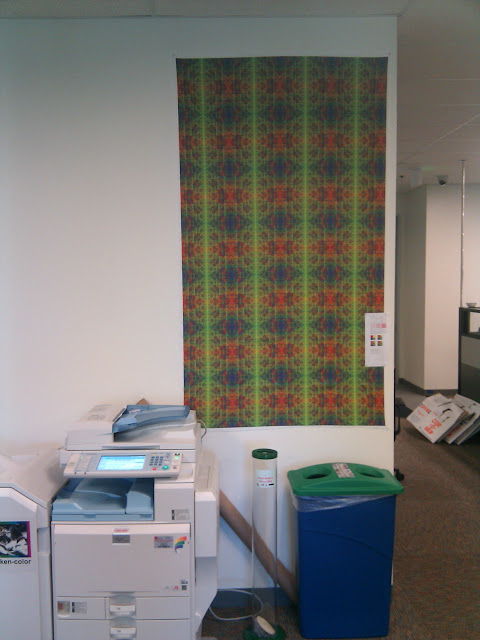Space-filling fabric is suitable for tiling any plain spots on your walls, or for topological application. For storage, fold into a moebius strip and store in a Klein Bottle.

(The “Hilbert Curve” is a recursive space-filling function. If we color the corners of each square instead instead of drawing lines between them, we get the pattern you see below.)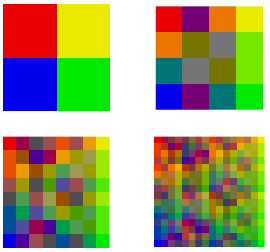Source code and images available at http://lunkwill.org/src/hilbert/.

Tuesday, June 29, 2010

Technical curriculum sucks

My wife just posted to her blog about how much she's enjoying the math classes she's taking at the community college.

She always did well in math, and had a friend/rival in elementary school who she always competed with to be the best in her math studies. Then in 6th grade, her teacher wouldn't let her move on to the fast track because she hadn't done enough homework to get an A, even though she was acing the tests.

Apart from feeling cheated, she was now also behind in the expected progression for technical fields. She studied English in college, and never got past college algebra.

Now she's back in school, thinking of doing a graduate degree in engineering. So she's at the community college, where she's supposed to take several years worth of prerequisites outside her final department, to get the math and science core she feels like she needs just to apply to a good grad school.

Likewise, my cousin loved astronomy in high school, where they had a neat research-oriented pilot program. Her project was to study historical photos of nebulas to see if expansion could be tracked over decades.

I encouraged her to take more when she got to college, but when she saw how many math and other science classes she'd have to take as prerequisites, she also decided to major in English.

One last related anecdote: I just met Sridhar Vembu last weekend. He found such a brain drain from the indian universities that he had trouble hiring for his indian software company. So he started a trade school. He takes 17-year-old poor kids with interest and a cursory hint of aptitude, and teaches them programming (while giving them a stipend and feeding them). The curriculum covers programming, english and math in that order of importance, and in that order of preference. The kids hate abstraction and math notation, but later appreciate it more after they've spent a few months solving practical problems in web programming. After 6-9 months, almost all of them start working for the company, and they do just as well as the college grads. As he puts it, "math is the new sanskrit".

Oh, and I believe he's taking about half and half girls/boys. (Except that more of the girls get recruited away by the colleges.)

You'd think that after 10 years of e-commerce, that shopping carts would be a solved problem. I just sent this feedback to spreadshirt.com.

Your shopping cart sucks. I bought a shirt from you through reddit.com, and I'm pretty sure I told it to order 3 shirts, but you only shipped and billed me for 1. So once I received it, I followed the link from the receipt to reorder. But clicking on the order number did nothing. My javascript console tells me that load_order() is undefined. I very nearly gave up at that point. So I went to spreadshirt.com directly, and clicked around until I found my order history there, and load_order() is defined from there. So somebody screwed up the javascript includes.
But it gets better. I ordered 5 shirts this time, looked for an "update" button and didn't find one, and clicked to continue the order. Only on the last page of the order process did I notice that the quantity was still at 1.

So I clicked on my shopping cart to back up the process and fix it. That showed me the item I was trying to order, and I clicked to proceed. But you were actually showing me the last page of the process again, so clicking committed the order... for one shirt.

Now on my fourth trip through the system, you at least gave me the option to cancel the order (thank you), and I once again repeated the process. And this time I found the "refresh" arrows next to the quantity. Protip: it's not actually that hard to have javascript code check the value of the quantity field when people click the button to go to the next page. You're throwing away orders and pissing people off when you expect your customers to find and click a button to tell your page something it should already know.

Finally, heading to the contact page, I get to enter a captcha (even though I'm already logged in) and type in a little tiny textbox to send you feedback, because you don't want to have to deal with the horrors of actually publishing an email address, even though you demand mine as part of the order process.

Friday, June 18, 2010

g++ error "does not exist in this scope" in karmic/lucid

g++-4.4 breaks backward compatibility for some stupid reason, so things that compiled just fine on earlier systems now break with errors like "stdout does not exist in this scope".

To fix, sudo apt-get install g++-4.2, then make sure your Makefiles or whatever are set to use g++-4.2 instead of just g++. You could probably also just apt-get remove g++-4.4, but I'm not sure if that'd break anything.

Thursday, June 17, 2010

OS statistics from wikimedia

It's surprisingly hard to find statistics on linux distribution popularity by version number.

But wikipedia to the rescue. Their squid logs break down user-agent string statistics by OS. Here's the May 2010 report:

http://stats.wikimedia.org/archive/squid_reports/2010-05/SquidReportOperatingSystems.htm

Linux seems to account for about 2% of all browsing. It looks like some browsers just report "Linux" as their OS, unfortunately. But among os-versioned browser strings, it looks like Ubuntu dominates linux distros by about 10:1, and almost all Ubuntu users are running either Lucid or Karmic (about 50/50).

indeed.

Saturday, June 12, 2010

Intuitively simple way to calculate sin and cos

A few years ago my brother in law asked how pi is calculated, and we found that it can be done as a reasonably intuitive algorithm, without any advanced math.

Ever since then, I've wondered if sines and cosines could also be calculated simply. Here's what I came up with. Please let me know if you know of a simpler way, where "simpler" means relying on fewer or more elementary mathematical principles.

Sines and cosines let us translate an angle in a right triangle into the length of one of is sides. But what is an angle? Well, on a unit circle, a 45 degree slice cuts off an arc that's 45/360ths the length of the circumference. We can cut that slice in half by finding the midpoint between the two ends of the arc, then extending that line out until it touches the edge of the circle. That slice represents 22.5 degrees. Since we have a point on the unit circle and we know its angle, the point's X and Y coordinates tell us the cosine and sine, respectively, of that angle. (And since the points are on a circle with a radius of 1, we know the hypotenuse of the right triangles is always of length 1).

Here's the same concept in pictures: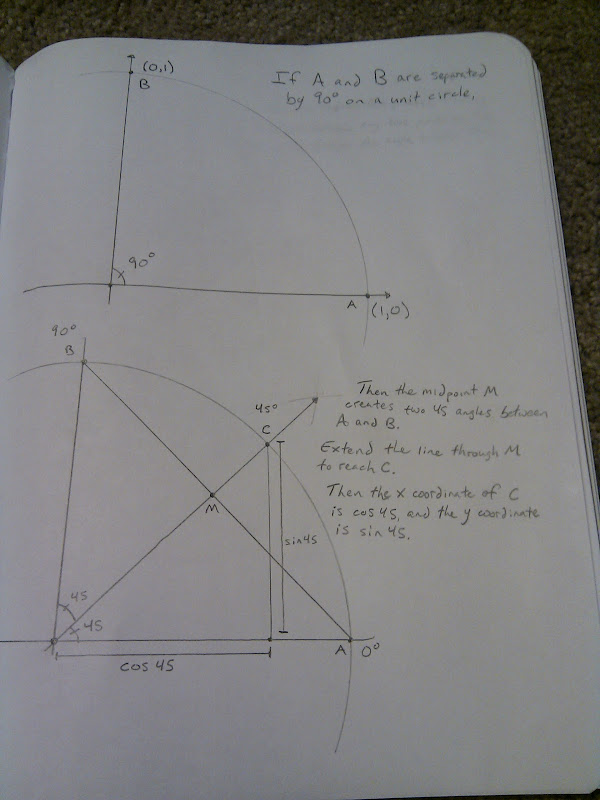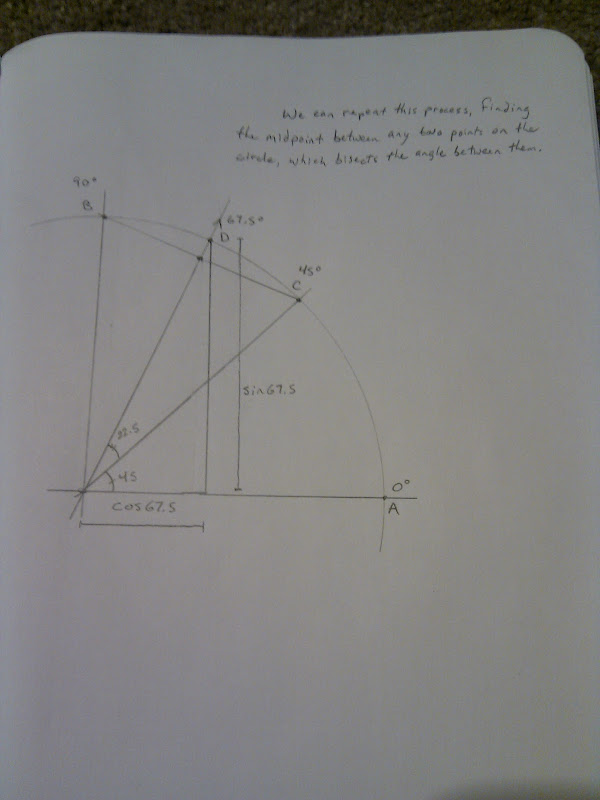And here's python code that starts with the two points shown in the pictures, and keeps finding midpoints between them (recursively) until it gets a close enough approximation to any angle you want.

#!/usr/bin/python
"""
Released into the public domain, 12 June 2010

This program calculates the sine and cosine of an angle in a geometrically
intuitive way.

Here's how it works:

Start with a unit circle and observe that the points (1,0) and (0,1) on the
circumference of the circle are separated by 90 degrees.

The midpoint between those two points, (0.5, 0.5), bisects that 90
degree angle, forming two 45 degree angles. We can extend the line from
the origin through (0.5, 0.5) until it reaches the circumference of the unit
circle (that is, until its distance from the origin is 1), and we get
(0.707, 0.707). Thus we know that the cosine and sine of 45 degrees are both
0.707.

Likewise, we can find the cosine and sine of 22.5 degrees by taking
the midpoint between (0.707, 0.707) and (1,0), then scaling the line until
it reaches the circumference. And we can find the cosine and sine of
67.5 degrees by taking the midpoint between (0.707, 0.707) and (0, 1).

We can continue to bisect angles until we get arbitrarily close to any
angle we choose. The allowable angular error is set below as MAX_THETA_ERROR.

Note that this method is agnostic to the angular units we use. If we start off
by telling the program that (0,1) and (1,0) are separated by an angle of 90,
then the program will return a result measured in degrees.

If instead we say that (0,1) and (1,0) are separated by pi/2, we'll get a
"""

import math

MAX_THETA_ERROR=0.01

def cos_sin(theta, x1,y1,theta1, x2,y2,theta2):
"""cos_sin returns the (cosine,sine) of a value within MAX_THETA_ERROR
units of theta, given two points (x1,y1) and (x2,y2) that live
at angular posisions theta1 and theta2 on the unit circle. theta,
theta1 and theta2 must all have the same kind of angular unit (degrees,
radians, etc.), but this function doesn't care what that unit is or
how many of them make up a circle.

theta2 must be greater than theta1, and (x1,y1) and (x2,y2) must be
points on the circumference of the unit circle, less than 180 degrees
apart.

for example, cos_sin(30.0, 0.0,1.0,0.0, 1,0,0.0,90.0) returns
(0.866089312575, 0.499889290387), which are the cosine and sine of 30.
"""

# Uncomment this to see the successive approximations
#print "target: %.2f. (%.2f, %.2f)=%.2f (%.2f,%.2f)=%.2f" % \
# (theta, x1, y1, theta1, x2, y2, theta2)

# if one of the points is close enough to theta, we're done!
if (abs(theta1 - theta) < MAX_THETA_ERROR):
return (x1,y1)
if (abs(theta2 - theta) < MAX_THETA_ERROR):
return (x2,y2)

# the midpoint is just the average of the two points
x_midpoint = (x1 + x2) / 2.0;
y_midpoint = (y1 + y2) / 2.0;

# scale (x_midpoint,y_midpoint) by 1/midpoint_length to get a point
# exactly 1 unit away from the origin
midpoint_length = math.sqrt(x_midpoint*x_midpoint + y_midpoint*y_midpoint)
x_midpoint = x_midpoint / midpoint_length
y_midpoint = y_midpoint / midpoint_length

# the midpoint bisects the angle between the two points
theta_midpoint = (theta1 + theta2) / 2.0;

# figure out which side of the midpoint our target value theta lives on,
# and bisect the extended midpoint with one of the original points to get
# closer to the target
if (theta >= theta_midpoint):
return cos_sin(theta, x_midpoint, y_midpoint, theta_midpoint, x2, y2, theta2)
else:
return cos_sin(theta, x1, y1, theta1, x_midpoint, y_midpoint, theta_midpoint)

angle = 30.0
(cosine, sine) = cos_sin(angle, 1.0,0.0,0.0, 0.0,1.0,90.0)
print "The cosine of %.2f is %.2f" % (angle, cosine)
print "The sine of %.2f is %.2f" % (angle, sine)

Thursday, June 03, 2010

Going from odometry to position in a two-wheeled robot

My robot has two drive wheels on which I have odometry (plus some casters that we'll ignore). I want to use the odometry from the wheel encoders to keep track of where I've gone since I started counting.

Let's say the right wheel moves b inches while the left wheel moved a inches during some time interval. Assume b > a. Then if we let the robot keep moving forever with those wheel speeds, the robot will make a big circle counterclockwise on the floor about some point X. We want to find X so that we can use a and b to find the new wheel positions on the floor.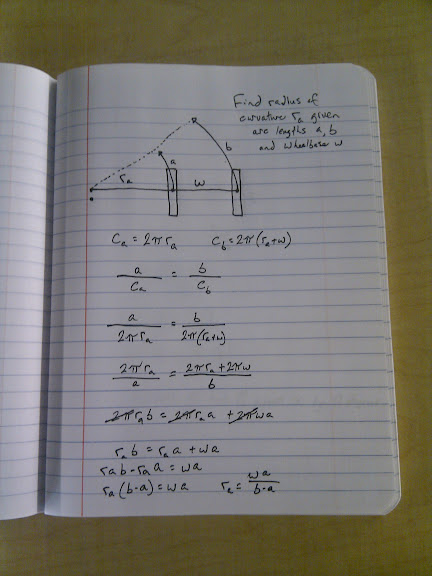We want to find ra, the distance from the left wheel to X.

We start by imagining the circumference of the circle the left wheel would travel in: ca. ca = 2*pi*ra. The right wheel's circle will have a radius of ra+w, where w is the wheelbase of the robot.

Since the two wheels are circling the same point on the floor with the axle always pointing toward the center of the circles, we know the distances a and b will constitute the same proportion of their respective circles. (If a goes all the way around its circle, b must have gone all the way around its circle too. Likewise if a goes 1/10th of the way around, etc.)

So we know that a/ca = b/cb, which is also the proportion of the circle we traveled (if a/ca = 0.10 then we've gone 10% around the circle). Substitute and solve and we get ra = w*a / (b-a).

Great! Now we know our radius of curvature.

Next, we want to find X, the actual point on the floor that we're circling. We start by finding theta, the angle we moved around the circle, by simply multiplying a/ca by 360 (for example, 0.10 * 360 = 36 degrees). If we want it in radians instead of degrees, it's even simpler, since multiplying by 2*PI cancels out the 2*PI in ca, leaving just a/ra.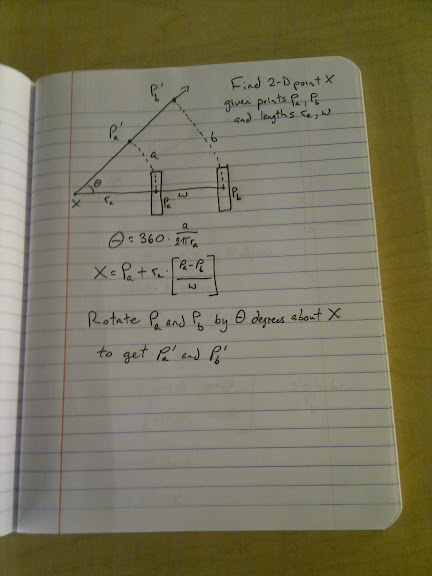We know that the point X is ra inches to the left of Pa, along a line which also passes through Pb. (X, Pa and Pb are 2-dimensional points). We get a vector from Pb to Pa with (Pa-Pb), make it length 1 by dividing by w, then multiply by ra so it's the right length to reach from Pa to X. Then we add it to Pa so that the vector ends at X.

Now we can find the updated wheel locations on the floor by rotating Pa and Pb by theta degrees around the point X. How do we do that? Well, we translate everything so that X is at the origin, then use a rotation matrix to rotate by theta, then translate back so that X is where it started: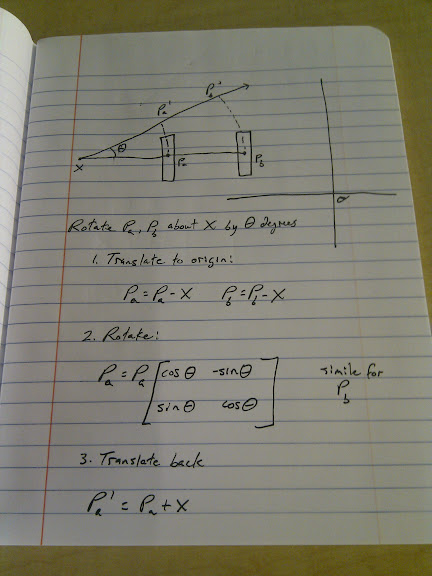Here's code that implements all of that (with saner variable names), plus the case where both wheels move the same amount):

// Just some math to turn wheel odometry into position updates
// Released into the public domain 3 June 2010

#include <stdio.h>
#include <math.h>
#include <stdlib.h>

#define PI (3.14159)

#define WHEELBASE (12.0)

// left wheel
double Lx = -WHEELBASE/2.0;
double Ly = 0.0;

// right wheel
double Rx = WHEELBASE/2.0;
double Ry = 0.0;

// given distances traveled by each wheel, updates the
// wheel position globals
void update_wheel_position(double l, double r) {

if (fabs(r - l) < 0.001) {
// If both wheels moved about the same distance, then we get an infinite
// radius of curvature. This handles that case.

// find forward by rotating the axle between the wheels 90 degrees
double axlex = Rx - Lx;
double axley = Ry - Ly;

double forwardx, forwardy;
forwardx = -axley;
forwardy = axlex;

// normalize
double length = sqrt(forwardx*forwardx + forwardy*forwardy);
forwardx = forwardx / length;
forwardy = forwardy / length;

// move each wheel forward by the amount it moved
Lx = Lx + forwardx * l;
Ly = Ly + forwardy * l;

Rx = Rx + forwardx * r;
Ry = Ry + forwardy * r;

return;
}

double rl; // radius of curvature for left wheel
rl = WHEELBASE * l / (r - l);

printf("Radius of curvature (left wheel): %.2lf\n", rl);

double theta; // angle we moved around the circle, in radians
// theta = 2 * PI * (l / (2 * PI * rl)) simplifies to:
theta = l / rl;

printf("Theta: %.2lf radians\n", theta);

// Find the point P that we're circling
double Px, Py;

Px = Lx + rl*((Lx-Rx)/WHEELBASE);
Py = Ly + rl*((Ly-Ry)/WHEELBASE);

printf("Center of rotation: (%.2lf, %.2lf)\n", Px, Py);

// Translate everything to the origin
double Lx_translated = Lx - Px;
double Ly_translated = Ly - Py;

double Rx_translated = Rx - Px;
double Ry_translated = Ry - Py;

printf("Translated: (%.2lf,%.2lf) (%.2lf,%.2lf)\n",
Lx_translated, Ly_translated,
Rx_translated, Ry_translated);

// Rotate by theta
double cos_theta = cos(theta);
double sin_theta = sin(theta);

printf("cos(theta)=%.2lf sin(theta)=%.2lf\n", cos_theta, sin_theta);

double Lx_rotated = Lx_translated*cos_theta - Ly_translated*sin_theta;
double Ly_rotated = Lx_translated*sin_theta + Ly_translated*sin_theta;

double Rx_rotated = Rx_translated*cos_theta - Ry_translated*sin_theta;
double Ry_rotated = Rx_translated*sin_theta + Ry_translated*sin_theta;

printf("Rotated: (%.2lf,%.2lf) (%.2lf,%.2lf)\n",
Lx_rotated, Ly_rotated,
Rx_rotated, Ry_rotated);

// Translate back
Lx = Lx_rotated + Px;
Ly = Ly_rotated + Py;

Rx = Rx_rotated + Px;
Ry = Ry_rotated + Py;
}

main(int argc, char **argv) {
if (argc != 3) {
printf("Usage: %s left right\nwhere left and right are distances.\n",
argv);
return 1;
}

double left = atof(argv);
double right = atof(argv);

printf("Old wheel positions: (%lf,%lf) (%lf,%lf)\n",
Lx, Ly, Rx, Ry);
update_wheel_position(left, right);
printf("New wheel positions: (%lf,%lf) (%lf,%lf)\n",
Lx, Ly, Rx, Ry);
}

Wednesday, June 02, 2010

atmel atmega32 avr-libc interrupt example

Edit: Fixed a bug in the example code where I tried to do _BV(foo|bar), which doesn't work at all. Changed them to (_BV(foo) | _BV(bar)). Also added a #define for _BV in case you don't already have it. I think this code is correct, but I haven't tried it.

I wanted to read a pair of quadrature encoders with my atmega32, so I needed to enable the INT0 and INT1 pins. Incidentally, if you don't mind throwing away half the resolution of your encoder, you can get away with using only one interrupt per encoder. (You put one of the pair of sensors on an interrupt, and the other one on a regular GPIO pin. You get interrupts half as often, and the other sensor tells you which way you moved).

Here's the code I used. The compiler complained about using obsolete avr-libc identifiers, but it seems to work fine.

#include <avr/interrupt.h>
#include <avr/signal.h>

// Just count the number of interrupts we see
int interrupt_counter = 0;
SIGNAL(SIG_INTERRUPT0) {
interrupt_counter++;
}

// I got lots of random resets until I added this handler.  So apparently you shouldn't enable
// an interrupt unless you have a handler installed.
SIGNAL(SIG_INTERRUPT1) {
interrupt_counter++;
}

And then in my init code:

#define _BV(x) (1 << (x))

// setup for servicing INT0, INT1 interrupt pins

// generate an interrupt on any logical change
MCUCR |= _BV(ISC10);
MCUCR &= ~_BV(ISC11);

MCUCR |= _BV(ISC00);
MCUCR &= ~_BV(ISC01);

// enable the interrupt pins
GICR |= _BV(INT1) | _BV(INT0);

// Set d2 and d3 to be inputs
DDRD &= ~(_BV(PD2) | _BV(PD3));

// Pullups off
PORTD &= ~(_BV(PD2) | _BV(PD3));